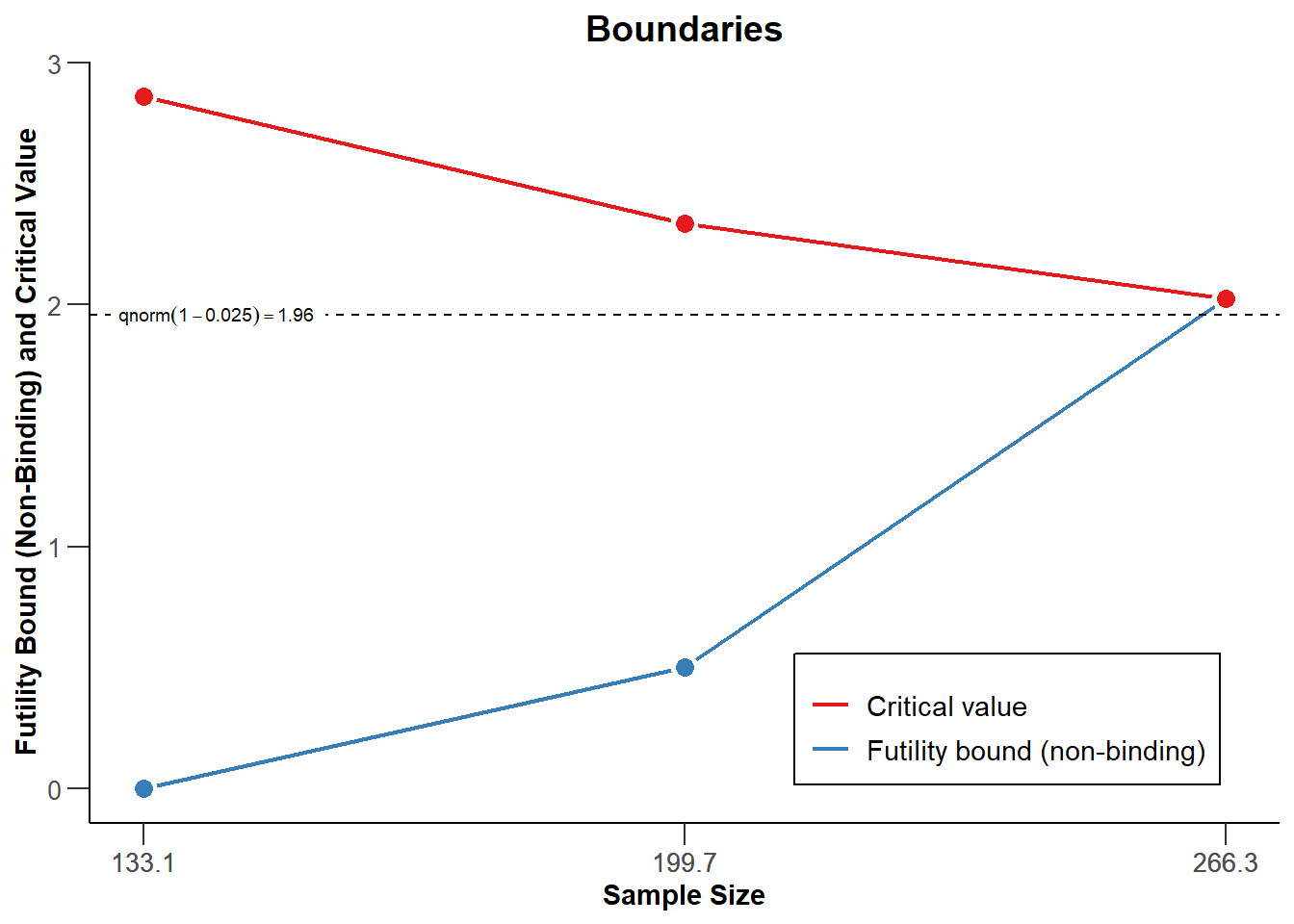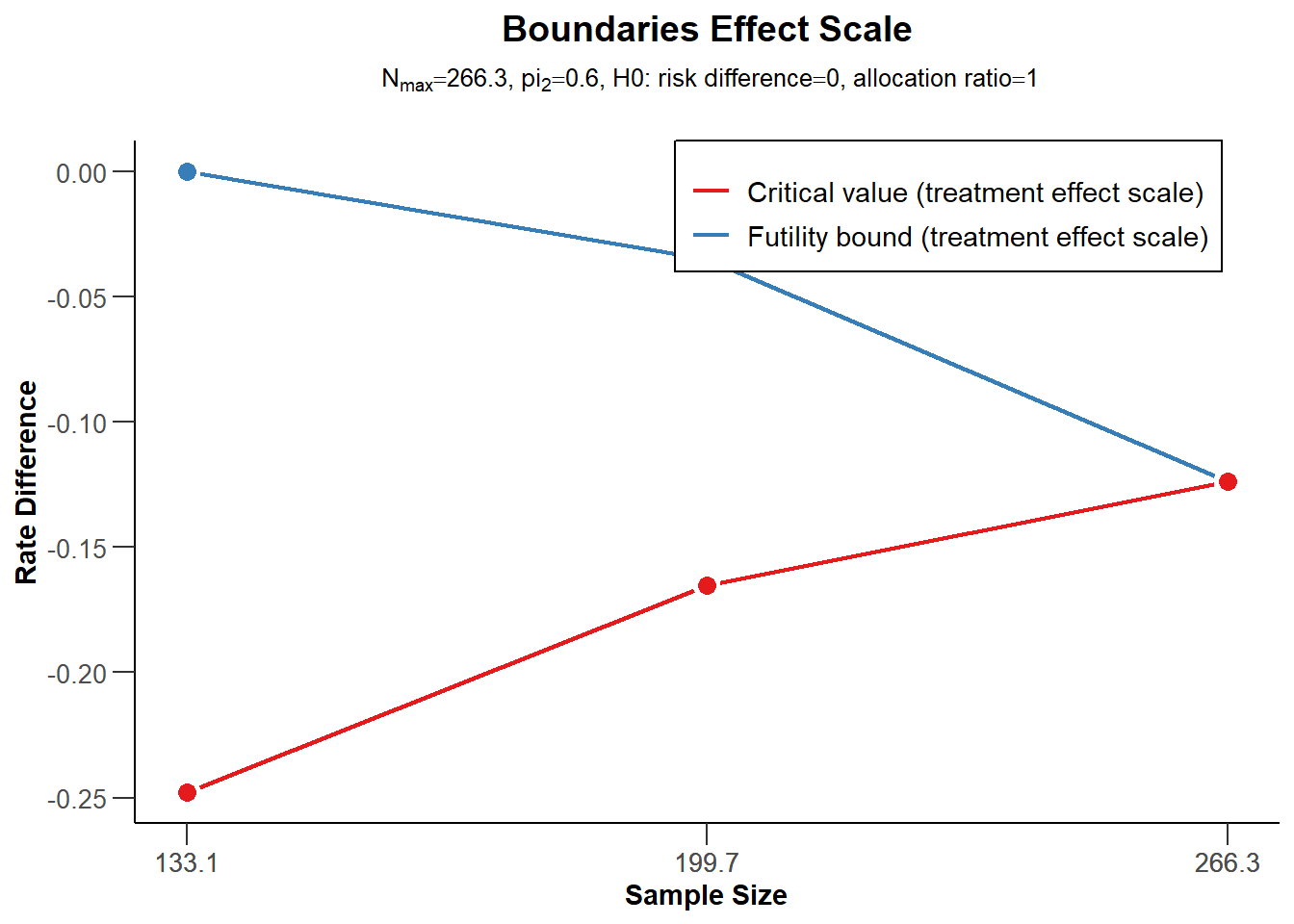# Summary

This R Markdown document provides an example for planning a trial with a binary endpoint using rpact. It also illustrates the use of ggplot2 for illustrating the characteristics of a sample size recalculation strategy. Another example for planning a trial with binary endpoints can be found here.

# 1 Designing a trial with binary endpoints

library(rpact)
packageVersion("rpact") # version should be version 2.0.5 or later
##  '3.1.1'

Suppose a trial should be conducted in 3 stages where at the first stage 50%, at the second stage 75%, and at the final stage 100% of the information should be observed. O’Brien & Fleming boundaries should be used with one-sided $$\alpha = 0.025$$ and non-binding futility bounds 0 and 0.5 for the first and the second stage, respectively, on the $$z$$-value scale.

The endpoints are binary (failure rates) and should be compared in a parallel group design, i.e., the null hypothesis to be tested is $$H_0:\pi_1 - \pi_2 = 0\,,$$ which is tested against the alternative $$H_1: \pi_1 - \pi_2 < 0\,.$$

## 1.1 Sample size calculation

The necessary sample size to achieve 90% power if the failure rates are assumed to be $$\pi_1 = 0.40$$ and $$\pi_2 = 0.60$$ can be obtained as follows:

dGS <- getDesignGroupSequential(informationRates = c(0.5,0.75,1), alpha = 0.025, beta = 0.1,
futilityBounds = c(0,0.5))
r <- getSampleSizeRates(dGS, pi1 = 0.4, pi2 = 0.6)

The summary() command creates a nice table for the study design parameters:

summary(r)
## Sample size calculation for a binary endpoint
##
## Sequential analysis with a maximum of 3 looks (group sequential design), overall
## significance level 2.5% (one-sided).
## The sample size was calculated for a two-sample test for rates
## (normal approximation),
## H0: pi(1) - pi(2) = 0, H1; treatment rate pi(1) = 0.4, control rate pi(2) = 0.6,
## power 90%.
##
## Stage                                         1      2      3
## Information rate                            50%    75%   100%
## Efficacy boundary (z-value scale)         2.863  2.337  2.024
## Futility boundary (z-value scale)             0  0.500
## Overall power                            0.2958 0.6998 0.9000
## Expected number of subjects               198.3
## Number of subjects                        133.1  199.7  266.3
## Exit probability for futility            0.0100 0.0056
## Cumulative alpha spent                   0.0021 0.0105 0.0250
## One-sided local significance level       0.0021 0.0097 0.0215
## Efficacy boundary (t)                    -0.248 -0.165 -0.124
## Futility boundary (t)                     0.000 -0.035
## Overall exit probability (under H0)      0.5021 0.2275
## Overall exit probability (under H1)      0.3058 0.4095
## Exit probability for efficacy (under H0) 0.0021 0.0083
## Exit probability for efficacy (under H1) 0.2958 0.4040
## Exit probability for futility (under H0) 0.5000 0.2191
## Exit probability for futility (under H1) 0.0100 0.0056
##
## Legend:
##   (t): treatment effect scale

Note that the calculation of the efficacy boundaries on the approximate treatment effect scale is performed under the assumption that $$\pi_2 = 0.60$$ is the observed failure rate in the control group and states the treatment difference to be observed in order to reach significance (or stop the trial due to futility).

## 1.2 Optimum allocation ratio

The optimum allocation ratio yields the smallest overall sample size and depends on the choice of $$\pi_1$$ and $$\pi_2$$. It can be obtained by specifying allocationRatioPlanned = 0. In our case, the optimum allocation ratio is 1 but calculated numerically, therefore slightly unequal 1:

r <- getSampleSizeRates(dGS, pi1 = 0.4, pi2 = 0.6, allocationRatioPlanned = 0)
r$allocationRatioPlanned ##  0.9999976 round(r$allocationRatioPlanned,5)
##  1

## 1.3 Boundary plots

The decision boundaries can be illustrated on different scales.

On the $$z$$-value scale:

plot(r, type = 1)On the effect size scale:

plot(r, type = 2)On the $$p$$-value scale:

plot(r, type = 3)